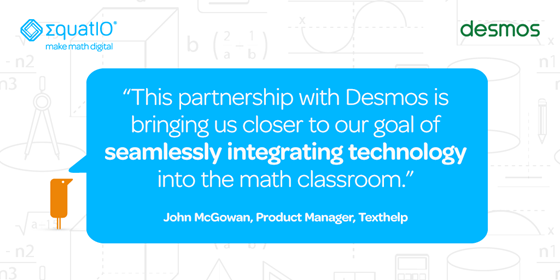# The Plot Thickens: EquatIO® now includes graphing from Desmos

Back in September, we announced a partnership with Desmos, which would introduce dynamic graphing into EquatIO. Today, Desmos graphing has been FULLY integrated into EquatIO across all platforms - EquatIO for Google, Windows, and Mac, and the web app, EquatIO mathspace.

For those that have never heard of Desmos, let’s take a step back. Desmos is the creator of the best-in-class HTML5 graphing calculator, which is already used by millions of math & STEM students around the world. The graphing calculator enables you to graph functions, plot data, evaluate equations, explore transformations, and so much more. Desmos has a ton of great resources at learn.desmos.com to dive deeper into the extensive functionality of their tool.By integrating Desmos’s robust graphing calculator into EquatIO, we’ve finally made EquatIO a one-stop-shop for making math and other STEM subjects digital. For teachers, this means you can easily create problem sets, worksheets, and quizzes without restrictions - incorporating formulas, geometric shapes, and graphs all in the same digital document, across all of your math & STEM lessons.For students, this means they now have one tool that offers them multiple means of representation, engagement, and expression - ideal for math & STEM classrooms that practice personalized learning or support accessible solutions for all students. For example, if a student is a visual learner, they can now use intuitive, dynamic graphing to visualize and explore a written equation that their teacher has asked them to evaluate.

What’s more, it is now even easier to create graphs in Desmos thanks to EquatIO’s prediction feature. Teachers and students alike can simply start typing the math function or expression they want to use in their graph and EquatIO will offer up suggestions. No tricky math languages or codes to learn.

Want to see the integration in action? Check out our how-to video of Desmos graphing in EquatIO:

Have you used Desmos in EquatIO? If so, please share tips and tricks in the comments section below!